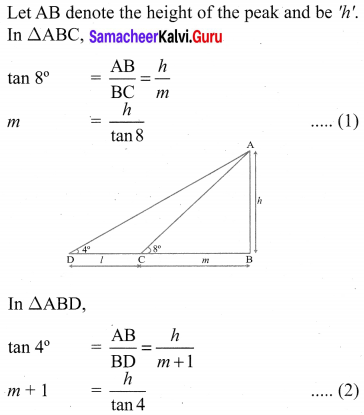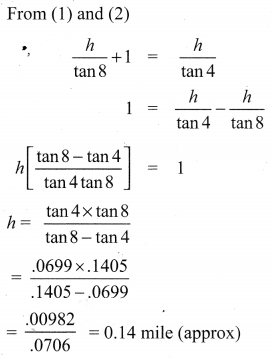# Samacheer Kalvi 10th Maths Solutions Chapter 6 Trigonometry Ex 6.2

## Tamilnadu Samacheer Kalvi 10th Maths Solutions Chapter 6 Trigonometry Ex 6.2

10th Maths Exercise 6.2 Samacheer Kalvi Question 1.
Find the angle of elevation of the top of a tower from a point on the ground, which is 30 m away from the foot of a tower of height 10$$\sqrt{3}$$ m.
Solution: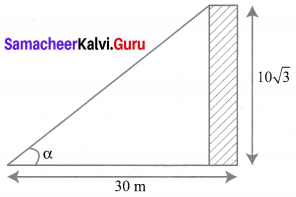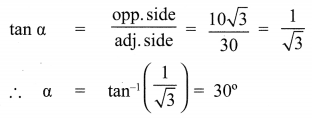Ex 6.2 Class 10 Samacheer Question 2.
A road is flanked on either side by continuous rows of houses of height 4$$\sqrt{3}$$ m with no space in between them. A pedestrian is standing on the median of the road facing a row house. The angle of elevation from the pedestrian to the top of the house is 30°. Find the width of the road.
Solution: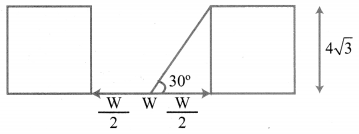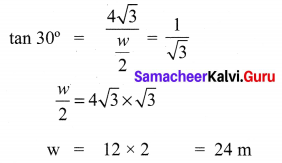10th Maths Trigonometry Exercise 6.2 Question 3.
To a man standing outside his house, the angles of elevation of the top and bottom of a window are 60° and 45° respectively. If the height of the man is 180 cm and if he is 5 m away from the wall, what is the height of the window? ($$\sqrt{3}$$ = 1.732)
Solution: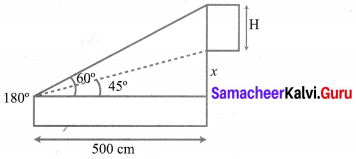Let ‘H’ be the fit of the window. Given that elevation of top of the window is 60°.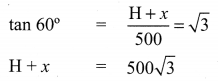Given that elevation of bottom of the window is 45°.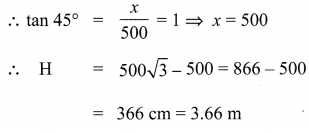∴ Height of the window = 3.66 m

Exercise 6.2 Class 10 Samacheer Kalvi Question 4.
A statue 1.6 m tall stands on the top of a pedestal. From a point on the ground, the angle of elevation of the top of the statue is 60° and from the same point the angle of elevation of the top of the pedestal is 40°. Find the height of the pedestal. (tan 40° = 0.8391, $$\sqrt{3}$$ = 1.732)
Solution: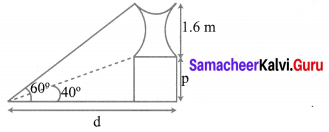Let ‘p’ be the fit of the pedestal and d be the distance of statue from point of cabs, on the ground.
Given the elevation of top of the statue from pf on ground is 60°.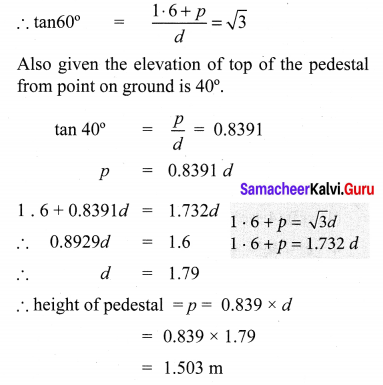10th Maths Exercise 6.2 Question 5.
A flag pole ‘h’ metres is on the top of the hemispherical dome of radius V metres. A man is standing 7 m away from the dome. Seeing the top of the pole at an angle 45° and moving 5 m away from the dome and seeing the bottom of the pole at an angle 30°. Find
(i) the height of the pole
Solution: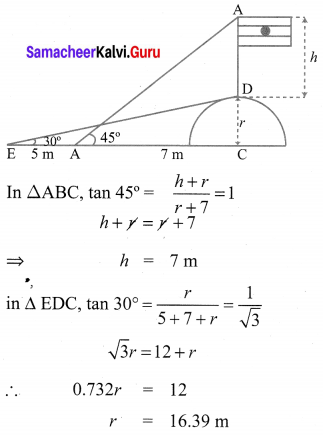10th Samacheer Kalvi Maths Trigonometry Question 6.
The top of a 15 m high tower makes an angle of elevation of 60° with the bottom of an electronic pole and angle of elevation of 30° with the top of the pole. What is the height of the electric pole?
Solution: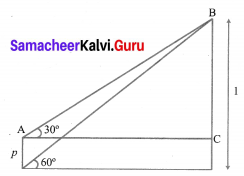Let BD be tower of height = 15 m
AE be pole of height = ‘p’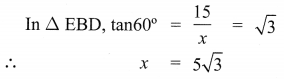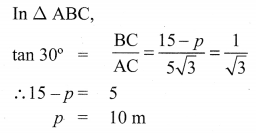10th Maths 6.2 Question 7.
A vertical pole fixed to the ground is divided in the ratio 1 : 9 by a mark on it with lower part shorter than the upper part. If the two parts subtend equal angles at a place on the ground, 25 m away from the base of the pole, what is the height of the pole?
Solution: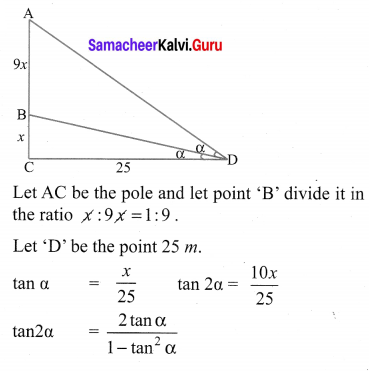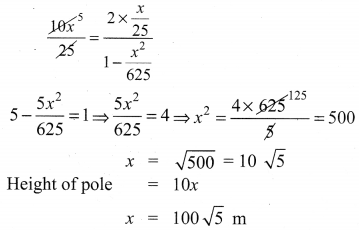Class 10 Ex 6.2 Solutions Question 8.
A traveler approaches a mountain on highway. He measures the angle of elevation to the peak at each milestone. At two consecutive milestones the angles measured are 4° and 8°. What is the height of the peak if the distance between consecutive milestones is 1 mile, (tan 4° = 0.0699, tan 8° = 0.1405).
Solution: